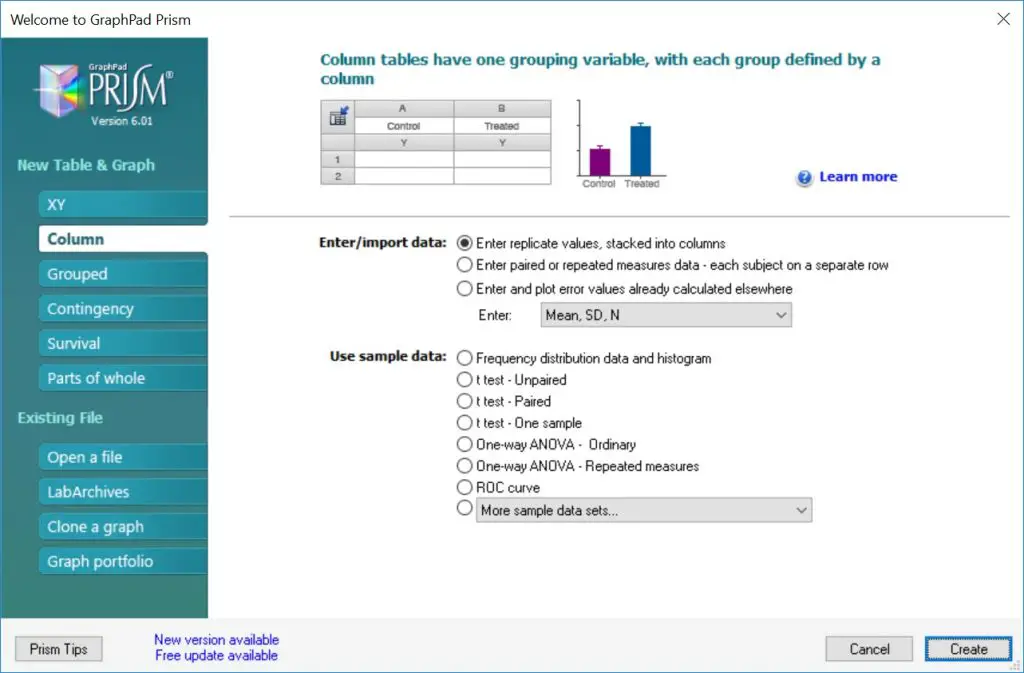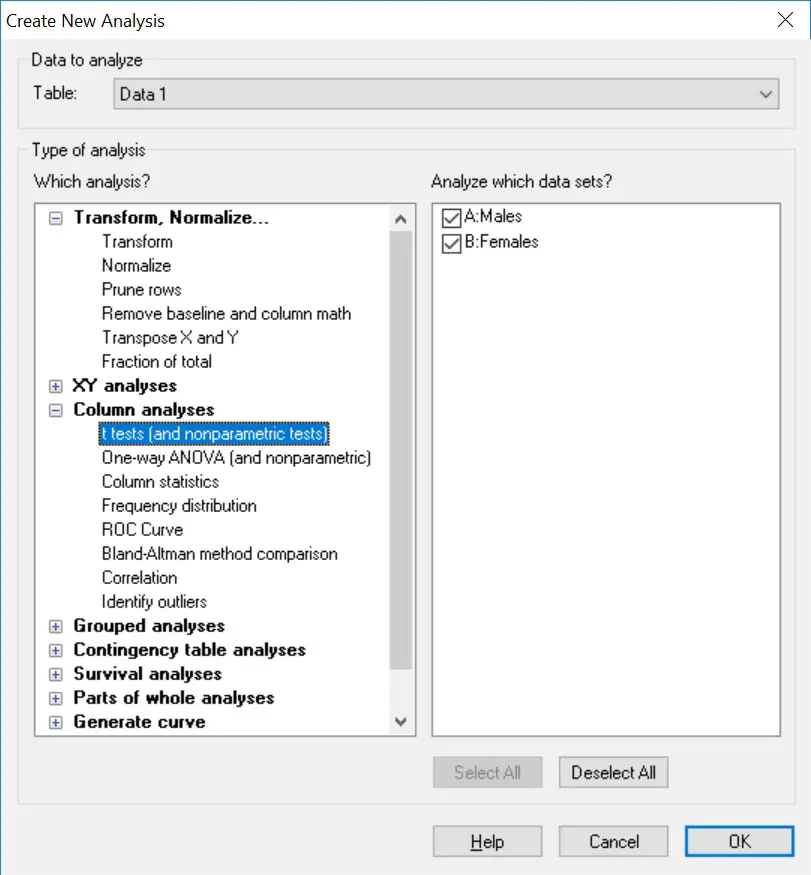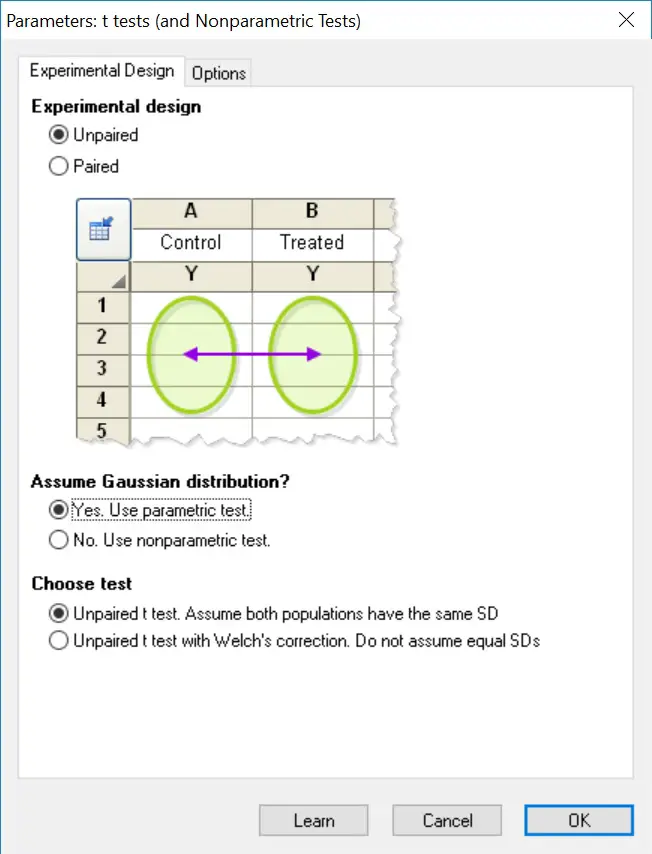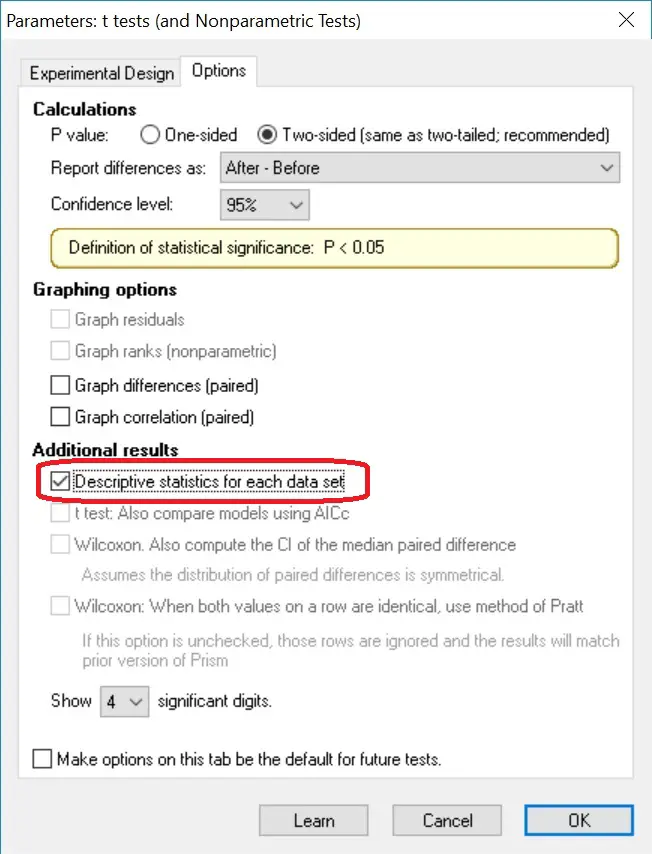How To Perform An Unparied T-Test In GraphPad Prism

In this guide, I will explain how to perform an unpaired (independent) T-test in GraphPad Prism. I will also show you how to interpret and report the results.

Assumptions

Before beginning it is important that your data satisfies the five assumptions of an independent t-test. Refer to our article on this by clicking here.

The data

To perform an unpaired (independent) T-test in GraphPad Prism you will need to enter two groups of data into separate columns. Upon opening GraphPad Prism, select the ‘Column’ type for the ‘New Table & Graph’ option. Then select ‘Enter replicate values, stacked into columns’ as the ‘Enter/import data’ choice.

Alternatively, you can go to ‘File > New > New Data Table & Graph …’.I will use a theoretical example of comparing heights between a group of males and females.  So each gender will have a separate column listing all of the height values.

“There is no difference in height between the males and females”.

And our alternative hypothesis will be:

“There is a difference in height between males and females”.

Performing the test

To perform an unpaired (independent) T-test, first go to ‘Insert > New Analysis …’.This will open a new window. Here you need to tell GraphPad which test to perform. Select the ‘t-tests (and nonparametric tests)’ analysis and make sure the two datasets are ticked on the right window. Click the ‘OK’ button.The next window will ask you to specify whether the t-test is an unpaired (independent) or paired (dependent) test. In this case, select the ‘unpaired’ option.

Next, you need to select whether the test will be a parametric or nonparametric test. Under ‘Assume Gaussian distribution’, select ‘Yes. Use parametric test’. This will ensure an unpaired T-test is performed.

The final option titled ‘Choose test’ is referring to whether your two datasets have equal variance. Select, ‘Unpaired t-test. Assume both populations have the same SD.If you want to change any other settings, such as the confidence level, go to the ‘Options’ tab. For our purpose we are going to leave everything in the default settings, but will select ‘Descriptive statistics for each data set’ so GraphPad will produce the mean and standard deviation for each group. Click ‘OK’ to perform the test.Output

The great thing about GraphPad Prism for statistical testing is that the output is very user friendly and self-explanatory. All you need to do is to refer to the ‘Unpaired t-test’ section.

• P value – The exact p-value.
• P value summary – A summary of the p-value as represented by asterisks. These are useful to signify the level of significance on graphs, for example.
• Significantly different? (P<0.05) – A straight yes or no answer for the unpaired t-test.
• One- or two-tailed P value? – Whether a one- or two-tailed unpaired t-test was performed.
• t, df – The t statistic and the degrees of freedom in the analysis.

GraphPad also gives you other descriptive information for each dataset (under the ‘How big is the difference?’ section) and an output for the F test (under the ‘F test to compare variances’ section) to determine if there is an equal variance of data between the two groups.

Interpretation

By looking at the ‘Significantly different? (P<0.05)’ output, a ‘Yes’ is given which essentially means that our results are significantly different from each other. The exact p-value is given next to ‘P-value’. In this case a p-value of < 0.0001 indicates a very significant difference, since this is less that our level of significance threshold of 0.05.

So, after performing the unpaired T-test in GraphPad we now know that there is a significant difference between the height of males and females in our experiment. In which case, we reject the null hypothesis and accept the alternative hypothesis.

Reporting

If we were to report the results of this unpaired T-test in a single sentence, we could write:

“On average males (181.1 ± 4.9 cm) were significantly taller than females (163.4 ± 5.1 cm), t(14) = 7.15, p < 0.0001.”

This includes the mean and standard deviation (this information can be found in the ‘Descriptive statistics’ sheet) for each group, as well as the t statistic, degrees of freedom and the all-important p-value.

GraphPad Prism version used: 6